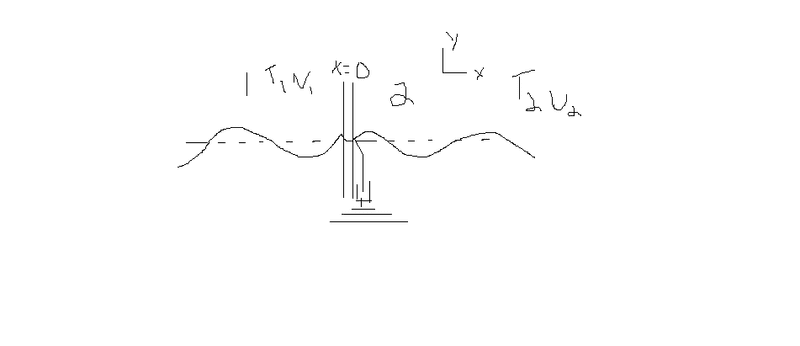# Boundary conditions for 2 ropes fixed to a massless ring with a damper

Redwaves
Homework Statement:
The boundary conditions for 2 ropes fixed to a massless ring with a massless damper
A wave comes from the left (rope 1) to the right (rope 2).
Relevant Equations:
##\psi_1 = Ae^{i(\omega t -k_1x)} + Re^{i(\omega t + k_1x)}##
##\psi_2 = Te^{i(\omega t -k_2x)}##
Hi,
I'm not quite sure if I'm correct. I need to find the boundary conditions for 2 ropes ##T_1 \mu_1, T_2 \mu_2## fixed at ##x=0## to a massless ring with a massless damper of force ##F_d - -bv_y##

Here what I think, since the ring and the damper is massless ##\sum F_y = 0##. Thus, ##-T_1 \frac{\partial \psi_1}{\partial x} + T_2 \frac{\partial \psi_2}{\partial x} -b \frac{dy}{dt} = 0##

Then, the height must be the same for the first rope, the ring and the second rope at ##x=0##, thus ##y_d = \psi_1(0,t) = \psi_2(0,t)##
Which mean, A + R = T, since ##Ae^{i(\omega t -k_1x)} + Re^{i(\omega t + k_1x)} = Te^{i(\omega t -k_2x)}## at x=0Last edited:

Mentor
Is there a diagram that goes with this problem? I'm having trouble getting all of the terms correct...

Redwaves
I did a diagram. Is it clear enough?

Mentor
I did a diagram. Is it clear enough?
Bwaaa. So the two ropes are co-linear, and there is a ring in the middle connecting them with some sort of damper? Is there really no diagram with the problem that you've been given?

Redwaves
It's exactly like that. I just drew it myself, but it's basically the same. Correct me if I'm wrong, but to find the boundary conditions, I don't think I need this diagram. Since, there are only 3 forces in the y direction and I know that the damper and the ring is massless.

The goal is the find that ##b = Z_1 - Z_2## to have ##R = 0##. However, I found ##R = -Z_1 + Z_2##. I'm pretty sure my boundary conditions is not correct. ##Z_i## are the impedance.

Last edited:
Redwaves
##-T_1 \frac{\partial \psi_1}{\partial x} + T_2 \frac{\partial \psi_2}{\partial x} -b \frac{dy}{dt} = 0##
##T = A + R##

With those conditions I get ##R = \frac{Z_1A - Z_2B - bA}{Z_1 + Z_2 +b}##
if ##b = Z_1 - Z_2##, ##R = 0##
This is the correct answer. But are my boundary conditions correct?

Homework Helper
Gold Member
##-T_1 \frac{\partial \psi_1}{\partial x} + T_2 \frac{\partial \psi_2}{\partial x} -b \frac{dy}{dt} = 0##
##T = A + R##

With those conditions I get ##R = \frac{Z_1A - Z_2B - bA}{Z_1 + Z_2 +b}##
if ##b = Z_1 - Z_2##, ##R = 0##
This is the correct answer. But are my boundary conditions correct?
This looks good to me, except I think the B in the numerator of R should be A.

Redwaves
If B in the numerator of R is A, I didn't get 0 if ##b = Z_1 - Z_2##

Homework Helper
Gold Member
If B in the numerator of R is A, I didn't get 0 if ##b = Z_1 - Z_2##

Can you show the individual steps of how you get ##R = \frac{Z_1A - Z_2B - bA}{Z_1 + Z_2 +b}## to reduce to ##R = 0## when ##b = Z_1 - Z_2##?